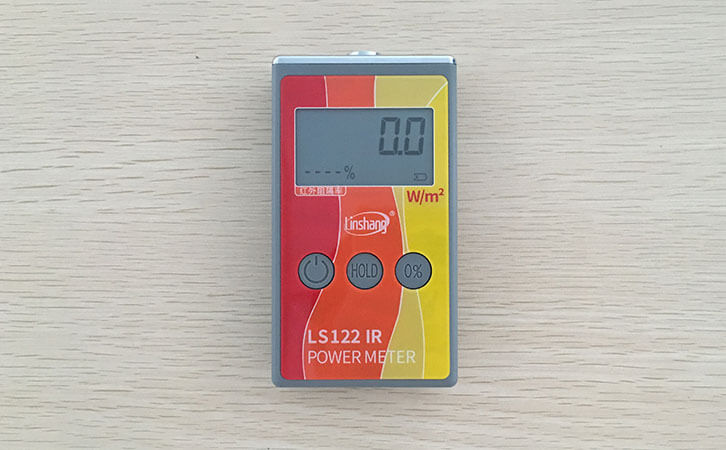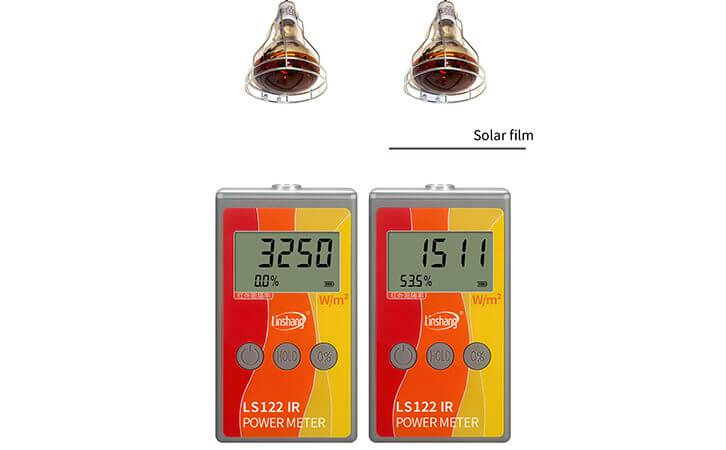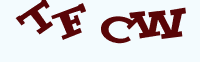# Linshang LS122 Infrared Power Meter

Time:2019/10/17 14:23:00 Browse:637

The Linshang LS122 infrared power meter is mainly used to measure the irradiance of infrared rays (ie, infrared power density) in various light sources and the infrared rejection rate of the test materials. Due to its compact size and intuitive display, it is widely used in the automotive window film industry to test the window film infrared barrier effect.In the actual use process, occasionally we will receive some customer feedbacks that why will it be a negative barrier value when using the Linshang LS122 infrared power meter? The infrared rejection rate reflects the rejection effect of the measured material on infrared rays.Even if the barrier effect is not good, the negative number is not likely to occur.What is the cause of that? We first learn how the infrared rejection value comes from the test principle of this infrared power meter.

## 1. Linshang LS122 infrared power metermeasurement principle

This infrared power meter is measured using the principle of transmittance. When no material is placed, the instrument will receive an original infrared intensity. After the material is placed, the instrument will receive an infrared light intensity value after passing through the material. By dividing the transmitted light intensity value by the original light intensity value, the infrared transmittance value of the tested material can be obtained. What is obtained by subtracting the transmittance value from 100% is the rejection ratio.## 2. The reason why the negative barrier value occurs when using the LS122 infrared power meter

It can be seen from the above measurement principle that the rejection ratio is equal to 100% minus the transmittance, and if the barrier ratio is negative, the transmittance value is greater than 100%. The transmittance of any material cannot be greater than 100%. What kind of operation will lead to such a result? In fact, during the test, when the original light intensity value is determined, the relative position of the infrared power meter and the light source changes, that is, the distance between the instrument and the light source is closer. Because the closer the source is to the instrument, the greater the intensity of the light received by the instrument. Therefore, when the distance between the light source and the infrared power meter becomes close, the infrared light intensity after the material is transmitted is larger than the original light intensity value. The light intensity after the material is transmitted is divided by the the original light intensity. You will get a transmittance value greater than 100%. Negative numbers occur when 100% is subtracted from a value greater than 100% to calculate the rejection rate.

When using the Linshang LS122 infrared power meter to test the infrared rejection rate of the material, be sure to not to move the instrument and the light source. Once the position changes, it will directly affect the measurement data. The above is an analysis of why the negative barrier value occurs when using the Linshang LS122 infrared power meter.

Enquiry

### EnquiryClick image refresh captcha
x